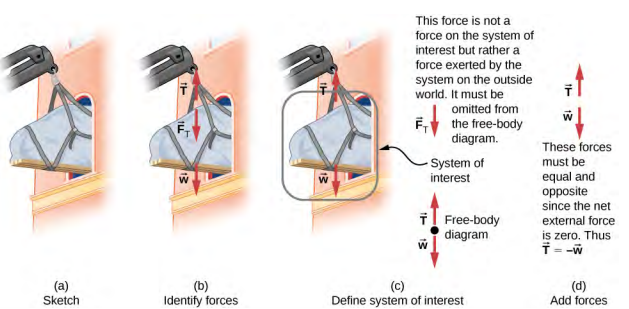$$\require{cancel}$$

# 6.2: Solving Problems with Newton's Laws (Part 1)

•• Contributed by OpenStax
• General Physics at OpenStax CNXFigure $$\PageIndex{6}$$: An Atwood machine and free-body diagrams for each of the two blocks.

## Strategy

We draw a free-body diagram for each mass separately, as shown in the figure. Then we analyze each diagram to find the required unknowns. This may involve the solution of simultaneous equations. It is also important to note the similarity with the previous example. As block 2 accelerates with acceleration a2 in the downward direction, block 1 accelerates upward with acceleration a1. Thus, a = a1 = −a2.

## Solution

1. We have $$For\; m_{1}, \sum F_{y} = T − m_{1}g = m_{1}a \ldotp \quad For\; m_{2}, \sum F_{y} = T − m_{2}g = −m_{2}a \ldotp$$(The negative sign in front of m2 a indicates that m2 accelerates downward; both blocks accelerate at the same rate, but in opposite directions.) Solve the two equations simultaneously (subtract them) and the result is $$(m_{2} - m_{1})g = (m_{1} + m_{2})a \ldotp$$Solving for a: $$a = \frac{m_{2} - m_{1}}{m_{1} + m_{2}}g = \frac{4\; kg - 2\; kg}{4\; kg + 2\; kg} (9.8\; m/s^{2}) = 3.27\; m/s^{2} \ldotp$$
2. Observing the first block, we see that $$T − m_{1}g = m_{1}a$$ $$T = m_{1}(g + a) = (2\; kg)(9.8\; m/s^{2} + 3.27\; m/s^{2}) = 26.1\; N \ldotp$$

## Significance

The result for the acceleration given in the solution can be interpreted as the ratio of the unbalanced force on the system, (m2 − m1)g, to the total mass of the system, m1 + m2. We can also use the Atwood machine to measure local gravitational field strength.

Exercise 6.3

Determine a general formula in terms of m1, m2 and g for calculating the tension in the string for the Atwood machine shown above.

# Contributors

• Samuel J. Ling (Truman State University), Jeff Sanny (Loyola Marymount University), and Bill Moebs with many contributing authors. This work is licensed by OpenStax University Physics under a Creative Commons Attribution License (by 4.0).